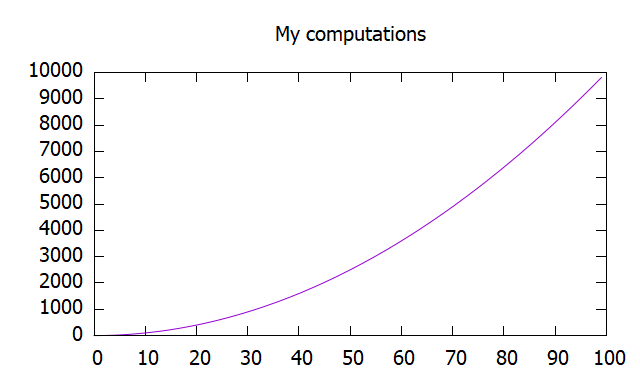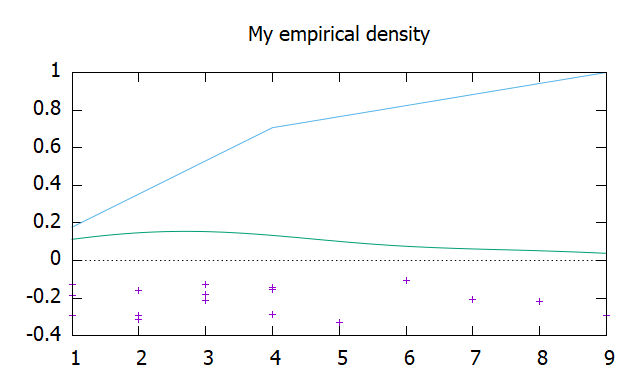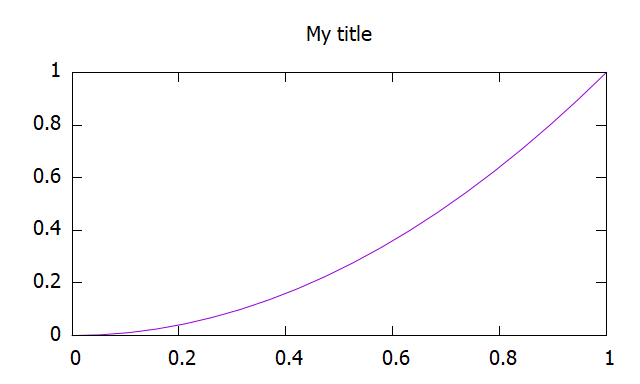# Crate preexplorer[−][src]

Expand description

Save data and have a glance at it with quick plots. Leave the detailed plotting to other interactive tools like gnuplot.

Do you have a costly process in Rust and want to save the data for postprocessing? Would you like to still have a basic glance to check it and leave fine-tuning of the plot for later? This is the crate for you!

# Philosophy

Rust is great at computing, making the perfect plot takes times and repetition. This repetitive process in search of the perfect plot should be done externally, and does not need Rust computing power. Therefore, once you achieve the data in Rust, save it, have a quick glance, and leave a simple gnuplot-script to start the fine tunning of your perfect plot.

# Remarks

All data will be saved under the folder “target\preexplorer\data” in the main directory. Plot scripts are saved under the foleder “target\preexplorer\plots”.

Recall that you will need to install gnuplot to use the crate at its full potential.

# Examples

```use preexplorer::prelude::*;
(0..100).map(|i| i * i)
.preexplore()
.set_title("My computations")
.plot("my_identifier")
.unwrap();```Check numerical simulations.

```use preexplorer::prelude::*;
use rand_distr::Exp1;
use rand::prelude::*;
let simulation_results: Vec<f64> = (0..100).map(|_| thread_rng().sample(Exp1)).collect();
pre::Density::new(simulation_results)
.set_title("Empirical Exponential 1")
.plot("my_identifier")
.unwrap();```Save some data (mostly numerical: matrices, simulation results and related errors, etc).

```use preexplorer::prelude::*;
let my_data = vec![0., 1.1, 0.001, 2., 2.3, 0.01, 3., 1.7, 0.02]; // Some data
let dimension = 2;
pre::Data::new(my_data, dimension)
.set_title("My title")
.plot_later("my_identifier")
.unwrap();```

Plot some function in a grid.

```use preexplorer::prelude::*;
use ndarray::Array;
let grid = Array::linspace(0., 1., 20);
let values = grid.iter().map(|x| x * x);
(grid.iter(), values).preexplore()
.set_title("My title")
.plot("my_identifier")
.unwrap();```Check out the gallery for more.

## Re-exports

`pub use self::traits::*;`

## Modules

Errors wrapper from writting data.

All you ussually need. Easily start preexploring you results.

Traits for easy use or self implmentation.

## Structs

Configuration for all basic options included.

Generic multi-dimensional data.

Comparison counter part of Density struct.

A type to a histogram: point cloud, probability density, cummulative probability distribution and/or bins.

Indexed sequence of values.

Comparison counter part of Heatmap struct.

Indexed sequence of values.

Indexed collection of histograms.

Comparison counter part of ProcessBin struct.

Indexed collection of values with a given error.

Comparison counter part of ProcessError struct.

Indexed collection of histograms, which will be represented as violins.

Comparison counter part of ProcessViolin struct.

Comparison counter part of Process struct.

Most basic explorable structure: a sequence of values.

Sequence of histograms normalize to represent a probability density function through bins.

Comparison counter part of SequenceBin struct.

Sequence of values with a given error.

Comparison counter part of SequenceError struct.

Sequence of violin plots.

Comparison counter part of SequenceViolin struct.

Comparison counter part of Sequence struct.

## Enums

A small collection of all possible styles.

## Constants

Path the data directory.

Path the plot scripts directory.

## Functions

Removes generated artifacts